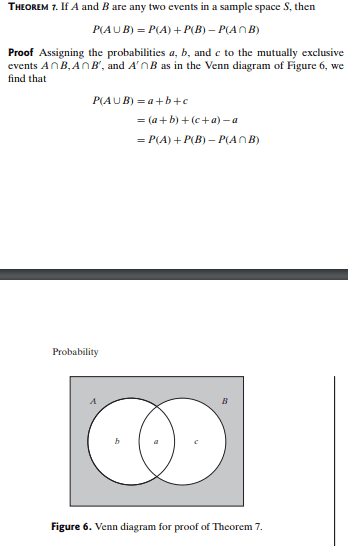# Statistics: Verifying a Probability Proof

• lema21

#### lema21

Homework Statement
Referring to figure 6, verify that P(A∩B')= P(A)-P(A∩B).
Relevant Equations
I have no idea how to start the solution and I've been looking on the web for similar questions but to no avail.•Delta2

I have no idea how to start the solution
doesn't pass the PF requirements to get assistance, but what the heck: you have a Venn diagram, so colour the appropriate areas !

Would drawing the diagrams for the LHS and RHS be enough to verify?

Would drawing the diagrams for the LHS and RHS be enough to verify?

It probably wouldn't qualify as a proof, but it would help show you why the probabilities are equivalent. Adding an algebraic argument in terms of a, b and c as in the proof you provided of Theorem 1, would count as a proof.

Would drawing the diagrams for the LHS and RHS be enough to verify?
Hint: if ##a = b - c## then ##a + c = b##.

I assume by B' you mean the compliment of B.

Looking at the diagram what is
P(A∩B')=?
P(A)=?
P(A∩B)=?
Give the answers in terms of a,b,c. Then you will see that the equation we want to prove transforms to something that algebraically is almost obvious.